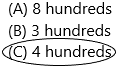# Texas Go Math Grade 2 Lesson 1.5 Answer Key Hundreds, Tens, and Ones

Refer to our Texas Go Math Grade 2 Answer Key Pdf to score good marks in the exams. Test yourself by practicing the problems from Texas Go Math Grade 2 Lesson 1.5 Answer Key Hundreds, Tens, and Ones.

## Texas Go Math Grade 2 Lesson 1.5 Answer Key Hundreds, Tens, and Ones

Explore

Write the number of hundreds, tens, and ones. Then draw a quick picture.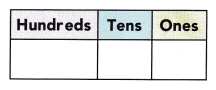FOR THE TEACHER • Read the following to children. Steven has 243 yellow blocks. How many hundreds, tens, and ones are in this number? Repeat for 423 red blocks.
The number of yellow blocks Steven has=243
Now we have to write the digits of place values.
The place value of 243 is: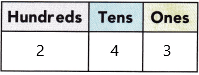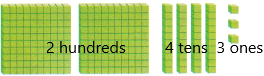2 is in the hundreds place
4 is in the tens place
3 is in the one’s place.
Likewise, we have to repeat for red blocks.
The number of red blocks steven has=423
Now we have to write the place values for 423:4 is in the hundreds place
2 is in the tens place
3 is in the one’s place

Math Talk
Mathematical Processes

Describe how the two numbers are alike. Describe how they are different.
Answer: The only difference is two numbers are place values.
In Mathematics, place value charts help us to make sure that the digits are in the correct places. To identify the positional values of numbers accurately, first, write the digits in the place value chart and then write the numbers in the usual and the standard form.
For example: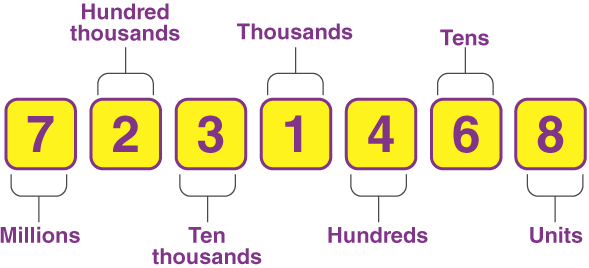Model and Draw

Write how many hundreds, tens, and ones there are in the model. What are two ways to write this number?Place value: Place value in Maths describes the position or place of a digit in a number. Each digit has a place in a number. When we represent the number in general form, the position of each digit will be expanded. Those positions start from a unit place or we also call it one’s position. The order of place value of digits of a number of right to left is units, tens, hundreds, thousands, ten thousand, a hundred thousand, and so on.
There are 2 blocks of hundreds it is equivalent to 200 so it was 2*100=200.
There are 4 blocks of tens it is equivalent to 10 so it was 4*10=40
There are 7 blocks of ones it is equivalent to 1 so it was 7*1=7
The total blocks:200+40+7 (expanded form)
The total blocks:247 (standard form)
Word form: Two hundred forty-seven.

Share and Show

Write how many hundreds, tens, and ones are in the model. Write the number in two ways.

Question 1.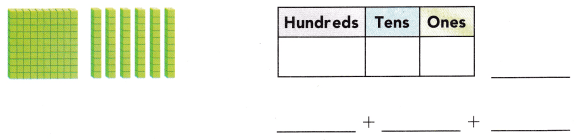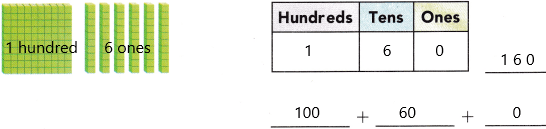Place value: Place value in Maths describes the position or place of a digit in a number. Each digit has a place in a number. When we represent the number in general form, the position of each digit will be expanded. Those positions start from a unit place or we also call it one’s position. The order of place value of digits of a number of right to left is units, tens, hundreds, thousands, ten thousand, a hundred thousand, and so on.
There is 1 block of hundreds it is equivalent to 100 so it was 1*100=300.
There are 6 blocks of tens it is equivalent to 10 so it was 6*10=60
There are 0 blocks of ones it is equivalent to 1 so it was 0*1=0
The total blocks:100+60+0 (expanded form)
The total blocks:160 (standard form)
Word form: One hundred sixty.

Question 2.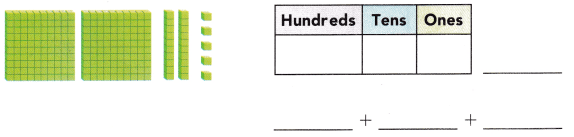Place value: Place value in Maths describes the position or place of a digit in a number. Each digit has a place in a number. When we represent the number in general form, the position of each digit will be expanded. Those positions start from a unit place or we also call it one’s position. The order of place value of digits of a number of right to left is units, tens, hundreds, thousands, ten thousand, a hundred thousand, and so on.
There are 2 blocks of hundreds it is equivalent to 100 so it was 2*100=200.
There are 2 blocks of tens it is equivalent to 10 so it was 2*10=20
There are 5 blocks of ones it is equivalent to 1 so it was 5*1=5
The total blocks:200+20+5 (expanded form)
The total blocks:225 (standard form)
Two hundred twenty-five (word form)

Question 3.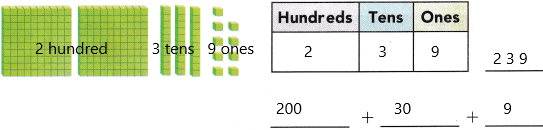Place value: Place value in Maths describes the position or place of a digit in a number. Each digit has a place in a number. When we represent the number in general form, the position of each digit will be expanded. Those positions start from a unit place or we also call it one’s position. The order of place value of digits of a number of right to left is units, tens, hundreds, thousands, ten thousand, a hundred thousand, and so on.
There are 2 blocks of hundreds it is equivalent to 100 so it was 2*100=200.
There are 3 blocks of tens it is equivalent to 10 so it was 3*10=30
There are 9 blocks of ones it is equivalent to 1 so it was 9*1=9
The total blocks:200+30+9 (expanded form)
The total blocks:239 (standard form)
Two hundred thirty-nine (word form)

Problem Solving

Write how many hundreds, tens, and ones are in the model. Write the number in two ways.

Question 4.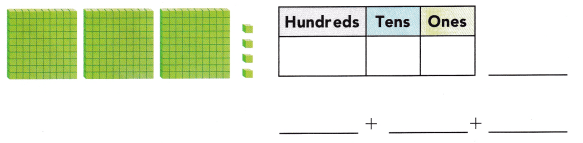Explanation:
Place value: Place value in Maths describes the position or place of a digit in a number. Each digit has a place in a number. When we represent the number in general form, the position of each digit will be expanded. Those positions start from a unit place or we also call it one’s position. The order of place value of digits of a number of right to left is units, tens, hundreds, thousands, ten thousand, a hundred thousand, and so on.
There are 7 blocks of hundreds it is equivalent to 100 so it was 3*100=300.
There are 5 blocks of tens it is equivalent to 10 so it was 0*10=0
There are 4 blocks of ones it is equivalent to 1 so it was 4*1=4
The total blocks:300+0+4 (expanded form)
The total blocks:304 (standard form)
Three hundred four (word form)

Question 5.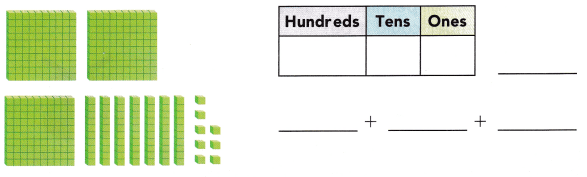Place value: Place value in Maths describes the position or place of a digit in a number. Each digit has a place in a number. When we represent the number in general form, the position of each digit will be expanded. Those positions start from a unit place or we also call it one’s position. The order of place value of digits of a number of right to left is units, tens, hundreds, thousands, ten thousand, a hundred thousand, and so on.
There are 7 blocks of hundreds it is equivalent to 100 so it was 3*100=300.
There are 5 blocks of tens it is equivalent to 10 so it was 7*10=70
There are 4 blocks of ones it is equivalent to 1 so it was 8*1=8
The total blocks:300+70+8 (expanded from)
The total blocks:378 (standard form)
Three hundred seventy-eight (word form)

Solve. Write or draw to explain.

Question 6.
H.O.T. A model for my number has 4 ones blocks, 5 tens blocks, and 7 hundreds blocks. What number am I?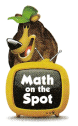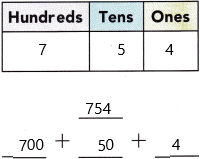Place value: Place value in Maths describes the position or place of a digit in a number. Each digit has a place in a number. When we represent the number in general form, the position of each digit will be expanded. Those positions start from a unit place or we also call it one’s position. The order of place value of digits of a number of right to left is units, tens, hundreds, thousands, ten thousand, a hundred thousand, and so on.
There are 7 blocks of hundreds it is equivalent to 100 so it was 7*100=700.
There are 5 blocks of tens it is equivalent to 10 so it was 5*10=50
There are 4 blocks of ones it is equivalent to 1 so it was 4*1=4

Question 7.
H.O.T. Multi-Step The hundreds digit of my number is greater than the tens digit. The one’s digit is less than the tens digit. What could my number be? Write it in two ways.
___________ + ___________ + ____________
_____________
Answer: In Mathematics, place value charts help us to make sure that the digits are in the correct places. To identify the positional values of numbers accurately, first, write the digits in the place value chart and then write the numbers in the usual and the standard form.
The 10 digits we used to represent the numbers are:
0    1    2    3    4    5    6    7    8     9
– In the hundreds digit the number should be greater than the tens digit. Assume that 9.
– The one’s digit should be less than the tens digit. Assume that 7.
– The tens digit should be less than the hundreds digit and greater than the ones digit. Assume that 8
The total number is 978
The expanded form of the number is 900+80+7.
The word form is nine hundred eighty-seven.

Question 8.
Representations Daniel has these bags of marbles. How many marbles does Daniel have?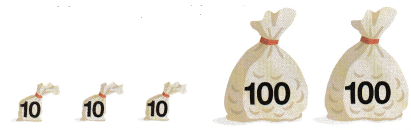(A) 110
(B) 320
(C) 230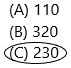Explanation: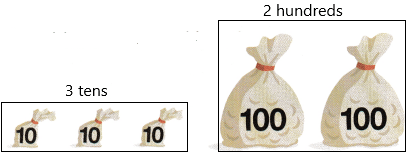There are 2 100’s bags and 3 10’s bags.
2 100’s is equivalent to 2*100=200
3 tens are equivalent to 3*10=30
So the place value will be 2 is hundreds place, 3 is in the tens place and 0 is in one’s place.

Question 9.
Analyze Gina uses pennies to buy a drum. The number of pennies she uses has 7 tens. Which number could it be?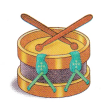(A) 274
(B) 167
(C) 728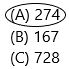Explanation:
The number of tens of pennies she uses=7
Now check out the options which number is having 7 in the tens place and this is the right option.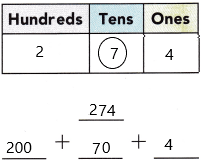Place value: Place value in Maths describes the position or place of a digit in a number. Each digit has a place in a number. When we represent the number in general form, the position of each digit will be expanded. Those positions start from a unit place or we also call it one’s position. The order of place value of digits of a number of right to left is units, tens, hundreds, thousands, ten thousand, a hundred thousand, and so on.

Question 10.
What is another way to write 2 hundreds, 3 ones, and 8 tens?
(A) 280
(B) 823
(C) 283
Explanation:We have to write the standard form.
The standard form of 2 hundreds, 3 ones, 8 tens is 283.
Place value in Maths describes the position or place of a digit in a number. Each digit has a place in a number. When we represent the number in general form, the position of each digit will be expanded. Those positions start from a unit place or we also call it one’s position. The order of place value of digits of a number of right to left is units, tens, hundreds, thousands, ten thousand, a hundred thousand, and so on.

Question 11.
TEXAS Test Prep Karen has 279 pictures. How many hundreds are in this number?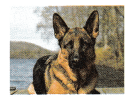(A) 9 hundred
(B) 2 hundred
(C) 7 hundredExplanation: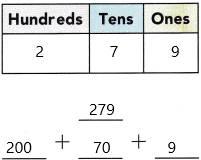The number of pictures Karen has=279
Here we have to write the place value of hundred.
Place value: Place value in Maths describes the position or place of a digit in a number. Each digit has a place in a number. When we represent the number in general form, the position of each digit will be expanded. Those positions start from a unit place or we also call it one’s position. The order of place value of digits of a number of right to left is units, tens, hundreds, thousands, ten thousand, a hundred thousand, and so on.
There are 2 blocks of hundreds it is equivalent to 100 so it was 2*100=200.
There are 7 blocks of tens it is equivalent to 10 so it was 7*10=70
There are 9 blocks of ones it is equivalent to 1 so it was 9*1=9

TAKE-HOME ACTIVITY • Say a 3-digit number, such as 546. Have your child draw a quick picture for that number.
Answer: Explanation is shown in the below representation.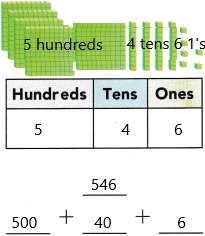Explanation:
Place value in Maths describes the position or place of a digit in a number. Each digit has a place in a number. When we represent the number in general form, the position of each digit will be expanded. Those positions start from a unit place or we also call it one’s position. The order of place value of digits of a number of right to left is units, tens, hundreds, thousands, ten thousand, a hundred thousand, and so on.
There are 5 blocks of hundreds it is equivalent to 100 so it was 5*100=500.
There are 4 blocks of tens it is equivalent to 10 so it was 4*10=40
There are 6 blocks of ones it is equivalent to 1 so it was 6*1=6
The total block: 500+40+6
The total block:546.

### Texas Go Math Grade 2 Lesson 1.5 Homework and Practice Answer Key

Write how many hundreds, tens, and ones are in the model. Write the number in two ways.

Question 1.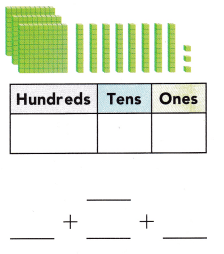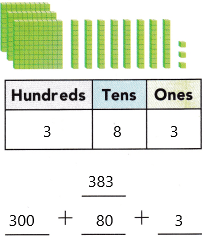3 blocks of hundreds and 3 blocks of ones and 8 blocks of tens.
Explanation:
Place value in Maths describes the position or place of a digit in a number. Each digit has a place in a number. When we represent the number in general form, the position of each digit will be expanded. Those positions start from a unit place or we also call it one’s position. The order of place value of digits of a number of right to left is units, tens, hundreds, thousands, ten thousand, a hundred thousand, and so on.

Question 2.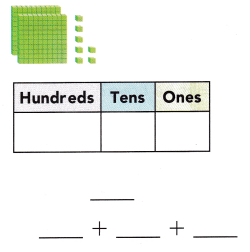2 blocks of hundreds and 7 blocks of ones and 0 tens.
Explanation:
Place value in Maths describes the position or place of a digit in a number. Each digit has a place in a number. When we represent the number in general form, the position of each digit will be expanded. Those positions start from a unit place or we also call it one’s position. The order of place value of digits of a number of right to left is units, tens, hundreds, thousands, ten thousand, a hundred thousand, and so on.

Problem Solving

Solve. Write or draw to explain

Question 3.
A model for my number has 6 one’s blocks, 2 tens blocks, and 8 hundred blocks. What number am I?
Explanation:
The number of one’s blocks=6
The number of tens blocks=2
The number of hundred blocks=8
Here we write the first hundred places, second tens place and third one’s place.The number is 826.

Question 4.
Multi-Step The hundreds digit of my number is less than the tens digit. The ones digit is greater than the tens digit. What could my number be?
_________ + _________ + ___________
__________
Answer: In Mathematics, place value charts help us to make sure that the digits are in the correct places. To identify the positional values of numbers accurately, first, write the digits in the place value chart and then write the numbers in the usual and the standard form.
The 10 digits we used to represent the numbers are:
0    1    2    3    4    5    6    7    8     9
– In the hundreds digit the number should be less than the tens digit. Assume that 1
– The one’s digit should be greater than the tens digit. Assume that 3.
– The tens digit should be greater than the hundreds digit and less than the ones digit. Assume that 2
The total number is 123
The expanded form of the number is 100+20+3.
The word form is one hundred twenty-three.

Lesson Check

Question 5.
The number of coins needed to buy a notepad has 3 tens. Which number could it be?
(A) 432
(B) 173
(C) 593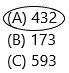Explanation:
3 tens are nothing but 3*10=30
The value of digits of 30:
3 is in the tens place
0 is in one’s place.
In option A 3 is in tens place so I prefer that option.

Question 6.
Tim has one pail of 100 coins. He has a second pail of 5 coins. He has the third pail of 40 coins. How many coins does he have?
(A) 154
(B) 451
(C) 145The number of one pail of coins Tim has=100
The number of the second pail of coins Tim has=5
The number of the third pail of coins Tim has=40
The total number of coins he has=X
Add the coins of 1st pail, second pail, and third pail
X=100+40+5
X=145
Therefore, the total coins are 145.

Question 7.
Sara wants to buy a toy. She needs 175 coins. Which model shows the number of coins?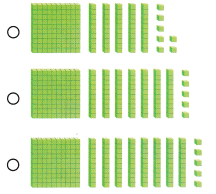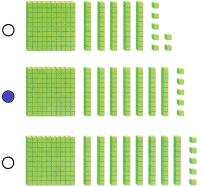Explanation: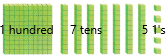The value of digits of 175:
1 is in the hundreds place
7 is in the tens place
5 is in one’s place.

Question 8.
Kevin has 438 marbles. How many hundreds are in this number?
(A) 8 hundred
(B) 3 hundred
(C) 4 hundred